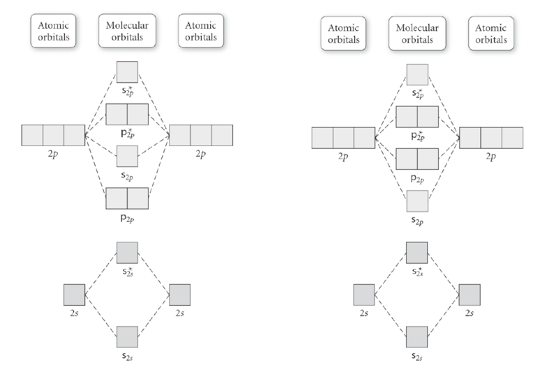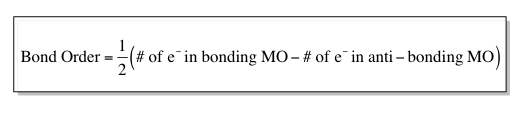Ch.10 - Molecular Shapes & Valence Bond TheoryWorksheetSee all chapters

# Molecular Orbital Theory

See all sections
Sections
Electron Geometry
Bond Angles
Hybridization
Molecular Orbital Theory
MO Theory: Heteronuclear Diatomic Molecules
Valence Shell Electron Pair Repulsion Theory
Delocalized Electrons
Molecular Geometry (IGNORE)
VSEPR Theory

Molecular Orbital Theory allows us to predict the distribution of electrons within a molecule. This allows us to predict properties such as bond order, magnetism and shape

###### Molecular Orbital (MO) Theory

Concept #1: Understanding Molecular Orbital Theory

Transcript

We've talked about one theory before, we've talked about VSEPR theory. Remember VSEPR means: valence shell electron pair repulsion and this has to do with molecular geometry. When we draw a compound, the lone pair electrons want more space for themselves, so they push all the other bonds away from them. When we're doing electronic geometry and molecular geometry and drawing Lewis dot structure, we're following VSEPR theory.
Now we're going to talk about the second type of theory, atomic theory or molecular orbital theory. We're going to say in the molecular orbital theory electrons are seen as being delocalized, or spread out over molecule instead of concentrated in a covalent bond.
Let's say we have a compound, hydrogen connected to Cl. Their forming a covalent bond with each other and realize that when it comes to a covalent bond, we have to see it as this, we have two forces basically fighting against each other.
We're going to have a bonding orbital is the region of high electron density between nuclei where a bond forms. The bond that we're forming is forming because we have bonding orbitals connecting together. We're going to say opposed to that, fighting against the bonding orbital, is the anti-bonding orbital. This is the region of zero electron density between the nuclei where a bond cannot form.
What this means is that every time we're forming a covalent bond between nonmetals, there are forces that want to keep the bond together and at the same time there are forces that exist that want nothing but to destroy that bond. These two bonding forces are basically fighting against one another. If bonding forces are greater, the bond forms. If anti-bonding forces are greater, the bond doesn't form. If they're equal the bond doesn't form. We want the bonding forces to always be greater so that a bond can be made.
How do we determine who's greater? The way we determine is by using these and these are our molecular orbital diagrams. I know it sucks, it looks crazy, but you have to memorize them. It's similar to the electron configuration that we learned earlier, except now we're doing molecular orbital electron configurations. But if you remember how to do electron configuration, this is very similar to it.
We're going to say the MO on the left side has atomic orbitals and molecular orbitals. The part that we're really concerned with are the molecular orbitals right here. These atomic orbitals on the left on the right to it, those come from the electron configurations which we use earlier. But it's the central parts of each of these MO diagrams that we're concerned with.
Here's the thing, we're going to say the one on the left deals with hydrogen, H2, to N2 on the periodic table. So hydrogen all the way to nitrogen, deals with the one on the left. The one on the right deals with O2, F2 and Ne2. Basically, for these molecular orbital diagrams, we're dealing with diatomic molecules. Remember, we know that there is only certain types of elements that can be diatomic molecules. Have No Fear Of Ice Cold Beer. That's the sentence we learned to remember the diatomic molecules.
Here's the thing, when we were doing MO theory we're going to treat all these elements whether they're diatomic or not, because neon normally isn't diatomic, but for the molecular orbital diagram, we treat it as though it's diatomic.
This is the way it's going to work. The way we look at this is, technically we start out with s1s because electron configuration begins with one s, but here this molecular orbital diagram shows us 2s at the beginning. This s here means sigma, so sigma 2s.
Here we have an s with a star, so this is sigma star 2s. When we see a star, that star signifies we have anti-bonding, so a star means anti-bonding. Here the 2s, molecular orbital is a bonding orbital and the sigma 2s molecular orbital is anti-bonding orbital.
We then go up to p, 2p. This p means pi. We have a pi 2p molecular bonding orbital, then we have sigma 2p bonding orbital, then we have a pi star, which means anti-bonding, orbitals and a sigma star 2p anti-bonding orbital. We're going to do just like we do with electron configuration to solve this.In MO theory, electrons are seen as being delocalized or spread out over a molecule instead of concentrated in a covalent bond.

Example #1: Use a MO diagram to write the electron configuration of each of the following:

C22-

Example #2: Use a MO diagram to write the electron configuration of each of the following:

F2+

###### Bond Order

Concept #2: Calculating Bond Order

Transcript

So remember, we say that there's Vesper theory and then there's molecular orbital theory, molecular orbital Theory uses these molecular orbital diagrams in order to write the electron configurations of these diatomic compounds, and we're going to say the molecular orbital diagram that we have, the one on the left and the one on the right, can be connected to a new idea bond order. Now, first remember that the one on the Left deals with H2 to N2 and then one on the on the right deals with O2, F2 and Ne2, and we're going to say bond order equals half times the number of electrons in bonding molecular orbitals minus the number of electrons in the anti-bonding molecular orbitals. Remember, the ones with stars are the anti-bonding molecular orbitals, the ones with no stars in them, those are our regular bonding molecular orbitals. Now, we're going to say based on our answers that we get from using this equation, our compound could be stable and exist or it can be unstable and not exist, we're going to say a bond order greater than 0 means that the compound is stable and exists. So, once, we write out the electron configuration using our molecular orbital diagrams, we would then use the bonding order formula, if the answer we get is greater than 0 that compound exists, if we get a bond order equal to 0 then that means the compound is unstable and it does not exist in reality and we're going to say in general the greater the bond order the stronger the bond, this ties into single bonds, double bonds, triple bonds.

So, remember let's say we have two carbons single bonded to each other, we have two carbons double bonded to each other and we have two carbons triple bonded to each other, we would say that the bond order of the two carbons that are single bonded is one, the bond order of the double bond is two because there's two bonds and the bond order of the triple bond is three, triple bonds are stronger than double bonds stronger than single bonds because the more bonds you put on top the more you layer the stronger the bond. So, this has to do a little bit with bond order, we're also going to say that the stronger the bond is the shorter the bond will be. So, although the triple bond is the strongest it's also the shortest. So, you've got to give up something to be that strong of a bond, we're going to say the single bond is the easiest to break but it is the longest and also remember, that if you have a single bond then you have one Sigma bond, if you have a double bond it's one Sigma and one pi, and if you have a triple bond it's one Sigma and two PI's, you always have one Sigma bond it forms the bond that connects the elements, the pi bonds are just overlapping orbitals that protect that Sigma bond, protect that single bond.

By calculating the bond order it is possible to determine the stability of the bonds within a molecule.Practice: Use a MO diagram to determine if the following compound exists or not.

O2 2- and B-